# 8.4.1 Radio Communication (Structured Questions)

Question 1:
Diagram 1.1 shows three types of electronic components used in a radio receiver.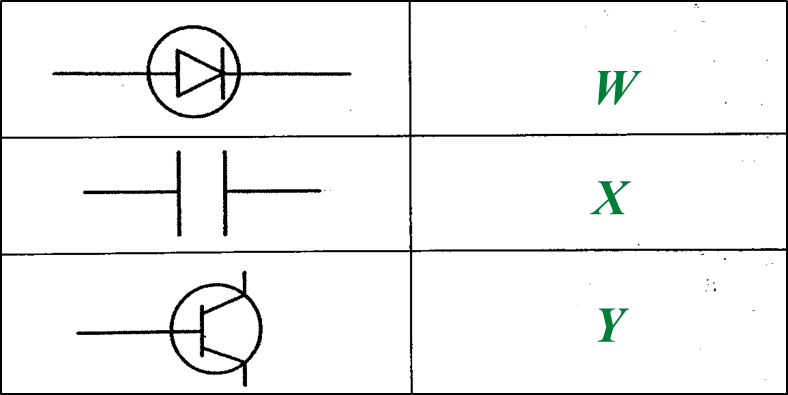Diagram 1.1

(a)
Name electronic components W and Y. [2 marks]

(b)
State the function of electronic component X. [1 mark]

(c)Diagram 1.2

(i)
Write any two electronic components, W, X or Y from Diagram 1.1 into the corresponding blocks provided in Diagram 1.2. [2 marks]

(ii)
What type of wave is received by T?
Mark (\/) your answer in the box provided in Diagram 1.3. [1 mark]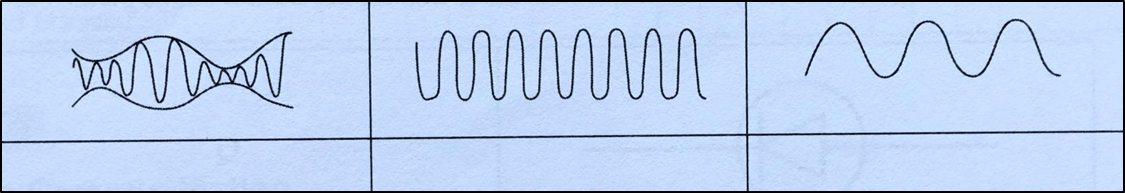Diagram 1.3

(a)
W: Diode
Y: Transistor

(b)
1. Stores electric charges and discharges them at regular intervals when required
2. To channel the flow of radio frequency carrier waves into the Earth.
3. When connected in parallel with the inductor, it determines the frequency of the radio wave that will be detected.

(any one)

(c)(i)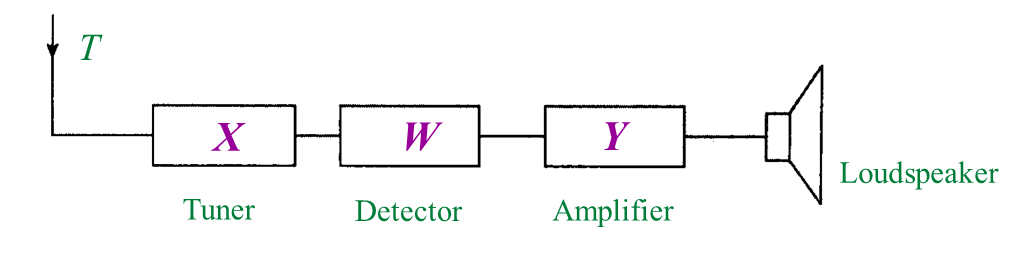(c)(ii)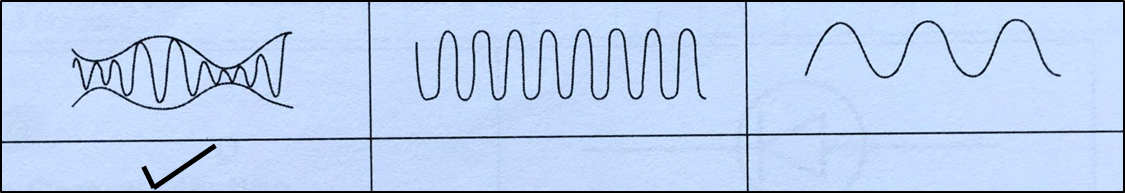Question 2:
Table 1 shows the symbols of electronic components.
(a) Complete Table 1 using the name of electronic components given. [3 marks]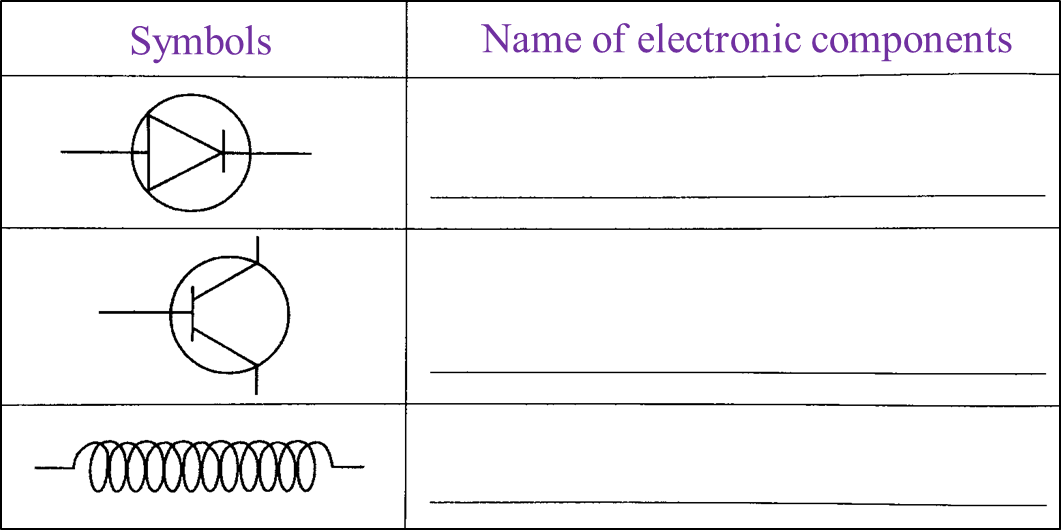Table 1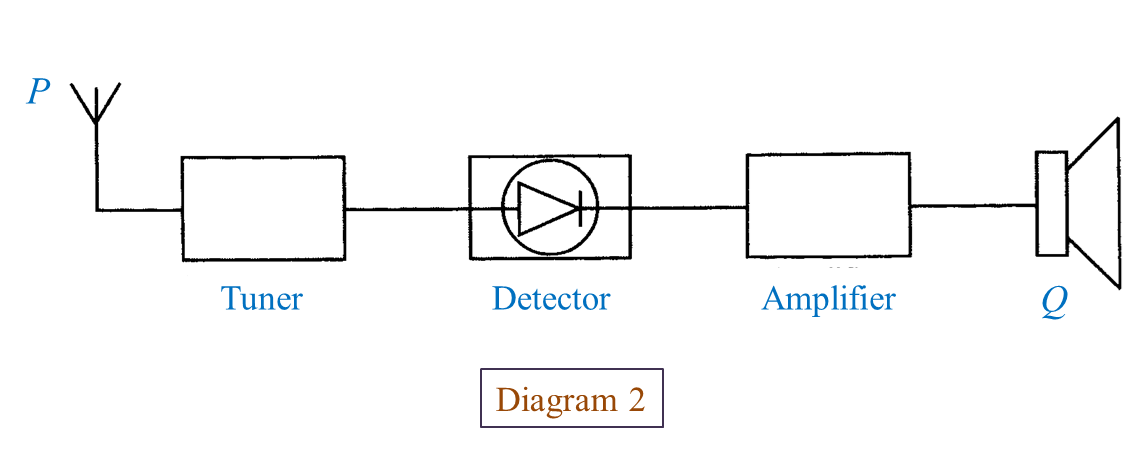(b) What is the function of P? [1 mark]

(c)
What is Q? [1 mark]

(d)
State the energy change that occurs at Q? [1 mark]

(a)(c)(i) Loudspeaker

(c)(ii) Electrical energy → sound energy

# 7.4.3 Practising Responsible Attitudes in the Disposal of Synthetic Polymers (Structured Questions)

Question 1:
Diagram 1 shows containers which are made of synthetic polymer.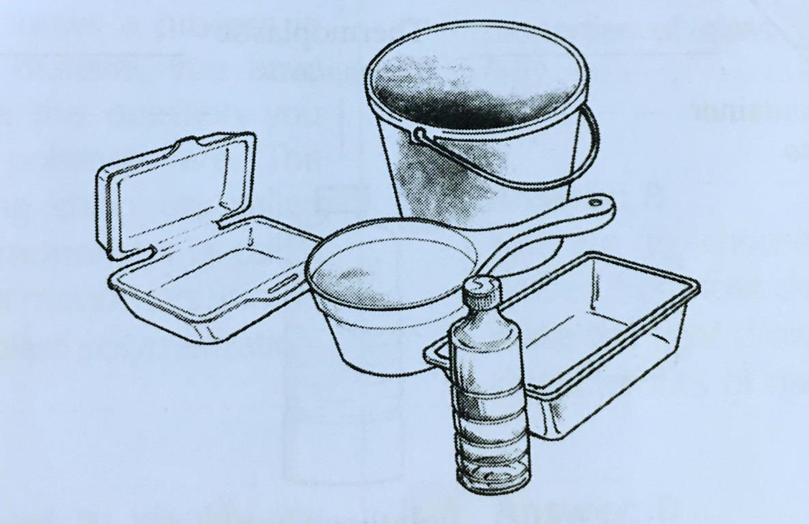Diagram 1

(a) Name the type of the synthetic polymer shown in Diagram 1. [1 mark]

(b)
State two characteristics of the synthetic polymer in Diagram 1. [2 marks]

(c)
What is the effect of improper disposal of the synthetic polymer in Diagram 1 on the environment? [1 mark]

(d)
State two correct ways to dispose of the synthetic polymer in Diagram 1 to preserve the environment. [2 marks]

(a)
Polythene or polyethene

(b)
- Soft when heated and hard when cooled
- Can be moulded more than once

(c)
Improper disposal of the synthetic polymers such as the burning of synthetic polymers releases toxic gases and acidic gases that cause air pollution and acid rain.

(d)
- Recycle or process again
- Burning in special incinerators which does not release toxic gases into the environment

# 7.4.2 Plastics (Structured Questions)

Question 1:
Table 1 shows the characteristics of plastic A and plastic B.Table 1

(a) State the type of plastic A and B. [2 marks]

(b)
State one example of plastic A and B. [2 marks]

(c)
Which plastic can be recycled? [1 mark]

(d)
Which plastic is suitable to make as the handle of an iron? [1 mark]

(a)
A: Thermoplastic
B: Thermosetting plastic (Thermoset)

(b)
A: Perspex
B: Bakelite

(c)
Perspex

(d)
Bakelite

# 7.4.1 Synthetic Polymers (Structured Questions)

Question 1:
Table 1.1 shows two types of product made of rubber.
(a) Complete Table 1.1. [4 marks]Table 1.1

(b) Table 1.2 shows the characteristics of plastic A and plastic B.Table 1.2

In Table 1.2, state the type of plastic A and plastic B. [2 marks]

(a)(b)# 15.2.3 Trigonometry, PT3 Focus Practice

Question 10:
A technician needs to climb a ladder to repair a street lamp as shown in Diagram below.(a) What is the length, in m, of the ladder?
(b) Find the height, in m, of the lamp post.

Solution: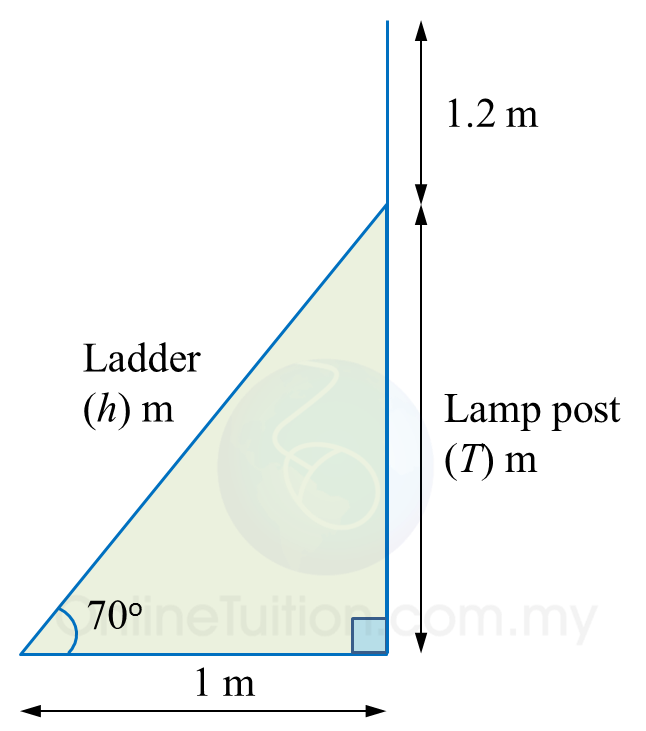(a)

(b)

# 15.2.2 Trigonometry, PT3 Focus Practice

          
Question 6:
 
In diagram below, AEC and BCD are straight lines. E is the midpoint of AC.(a) find the value of tan x.
 
(b) Calculate the length, in cm, of BD.  
 
 Solution:

       

          
Question 7:
 
In diagram below, T is the midpoint of the line PR.(a) Find the value of tan xo.
 (b) Calculate the length, in cm, of PQ.
 
 Solution:
               

 
 
          
Question 8:
 
In diagram below, ABE and DBC are two right-angled triangles ABC and DEB are straight lines.(a) Find the value of tan xo.
 (b) Calculate the length, in cm, of DE.
 
 Solution:
               

 
 

Question 9:
Diagram below shows a vertical pole, PQ. At 2.30 p.m. and 5.00 p.m., the shadow of the pole falls on QR and QS respectively.Calculate
(a) the height, in m, of the pole.
(b) the value of w.

Solution:
(a)

(b)

# 15.2.1 Trigonometry, PT3 Focus Practice

 
 
15.2.1 Trigonometry, PT3 Focus Practice
 

 
Question 1:
 
Diagram below shows a right-angled triangle ABC.It is given that    $\mathrm{cos}{x}^{o}=\frac{5}{13}$   , calculate the length, in cm, of AB.
 
 

Solution:
     

 
 
 
 
 
Question 2:
 
In the diagram, PQR and QTS are straight lines.It is given that $\mathrm{tan}y=\frac{3}{4}$ , calculate the length, in cm, of RS.
 
 

Solution:
     

 
 
 
 
 
Question 3:
 
In the diagram, PQR is a straight line.It is given that   $\mathrm{cos}{x}^{o}=\frac{3}{5}$  , hence sin yo =
 
 
 
 
Solution:
 
 

 
 
 

 
 
 
 
 
Question 4:
 
Diagram below consists of two right-angled triangles.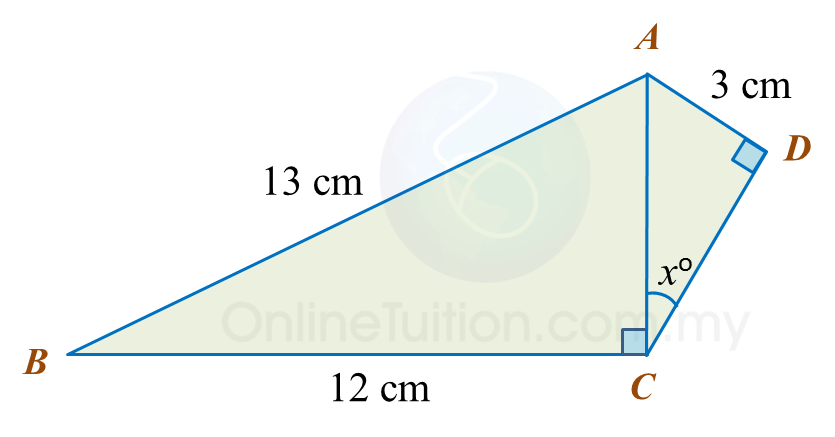Determine the value of cos xo.
 
 

Solution:
     
 

 
 
 
 
 
Question 5:
 
Diagram below consists of two right-angled triangles ABE and DBC.
 
ABC and EBD are straight lines.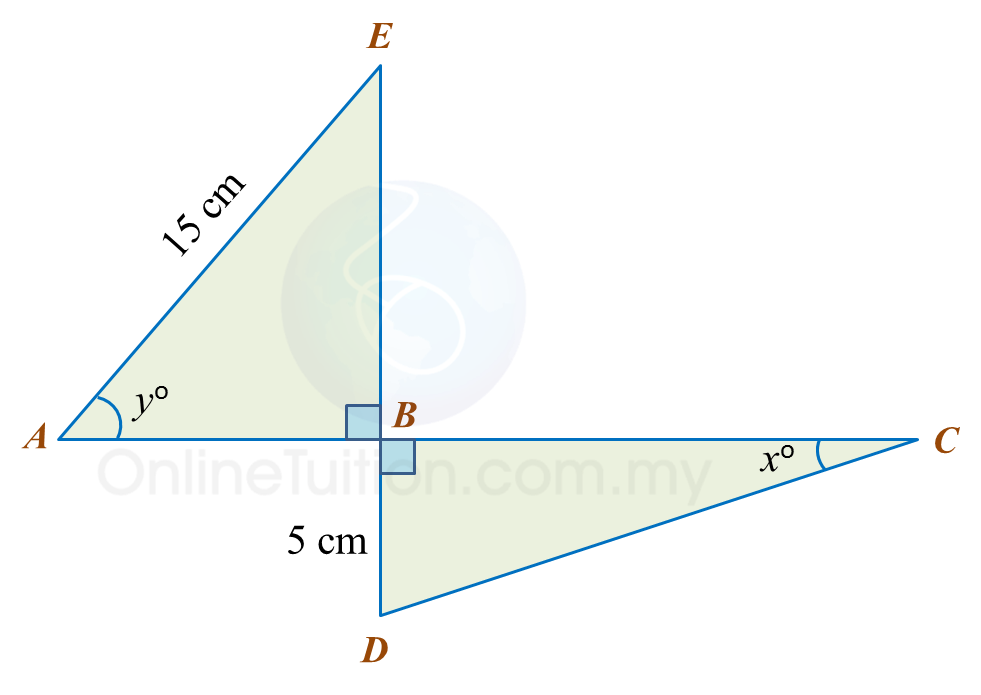It is given that
 
(a)  Find the value of tan xo.
 
(b)   Calculate the length, in cm, of ABC.
 
 

Solution:
 
(a)
 
   
 
 
 
(b)
 
   
 

 
 

# 15.1 Trigonometry

15.1 Trigonometry

15.1.1 Trigonometrical Ratios of an Acute Angle

1.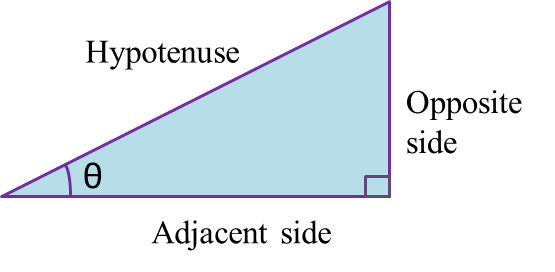2.   Hypotenuse is the longest side of the right-angled triangle which is opposite the right angle.
3.   Adjacent side is the side, other than the hypotenuse, which has direct contact with the given angle, θ.
4.   Opposite side is the side which is opposite the given angle, θ.
5.   In a right-angled triangle,

6.
When the size of an angle θ increases from 0o to 90o,

· sin θ increases.
· cos θ increases.
· tan θ increases.

7.   The values of sin θ, cos θ and tan θ remain the same even though the size of the triangle has changed.
Example: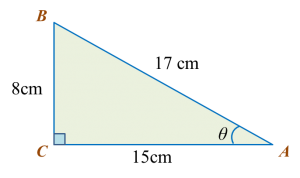Find the sine, cosine and tangent of the give angle, θ, in triangle ABC.

Solution:
$\begin{array}{l}\mathrm{sin}\theta =\frac{BC}{AB}=\frac{8}{17}\\ \mathrm{cos}\theta =\frac{AC}{AB}=\frac{15}{17}\\ tan\theta =\frac{BC}{AC}=\frac{8}{15}\end{array}$

15.1.2  Values of Tangent, Sine and Cosine
1.   The values of the trigonometric ratios of 30o, 45o and 60o (special angle) are as below.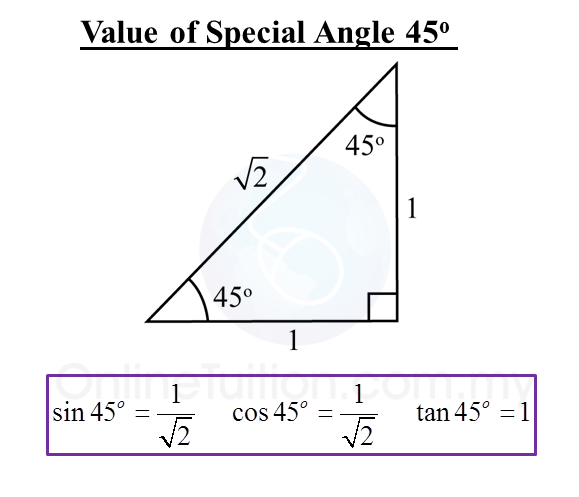2.   1 degree is equal to 60 minutes.
1o= 60’

3.   A scientific calculator can be used to find the value of the sine, cosine or tangent of an angle.
Example:
sin 40.6o = 0.5954

 Calculator Computation     Press [sin] [40.6] [=] 0.595383839
4.   Given the values of sine, cosine and tangent, we can find the angles using a scientific calculator.
Example:
tan x = 1.7862
x = 67o30’

 Calculator Computation Press [shift] [tan][1.7862]  [=][o’’’]  67o30’

# 14.2.3 Ratio, Rates and Proportions II, PT3 Focus Practice

Question 10:
Diagram below shows the distance from Town P to Town Q and Town Q to Town R.(a) Rahim rode his bicycle from Town P at 9.00 a.m. and took 2 hours to reach Town Q.
What is the speed, in km/h, of the bicycle?
(b) Rahim took 30 minutes rest at Town Q and continued his journey to Town R three times faster than his earlier speed.
State the time he reached Town R.

Solution:
(a)

(b)Question 11:
Diagram below shows a trailer travelling from a factory to location P and location P to location Q. The trailer departs at 8.00 a.m.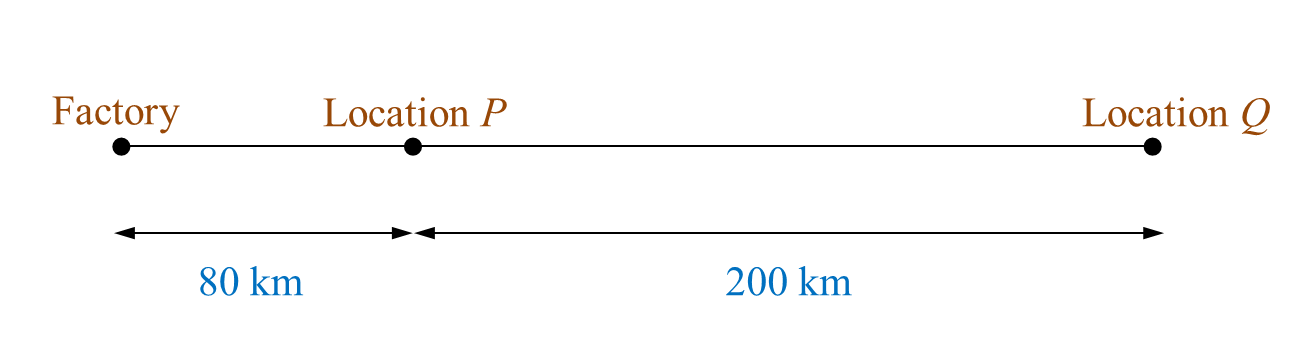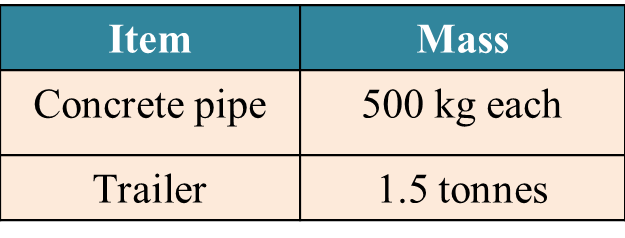(a) Based on the Table, calculate the total mass, in tonne, of the trailer and its load.
(b) The trailer arrived at location P at 10.00 a.m. and it stopped for 1½ hours to unload half of the concrete pipes. The trailer then continued its journey to location Q two times faster than its earlier speed. State the time, the trailer reached at location Q.

Solution:
(a)

(b)Question 12:
Diagram below shows travel information of Jason and Mary from Town A to Town B. Jason drives a lorry while Mary drives a car.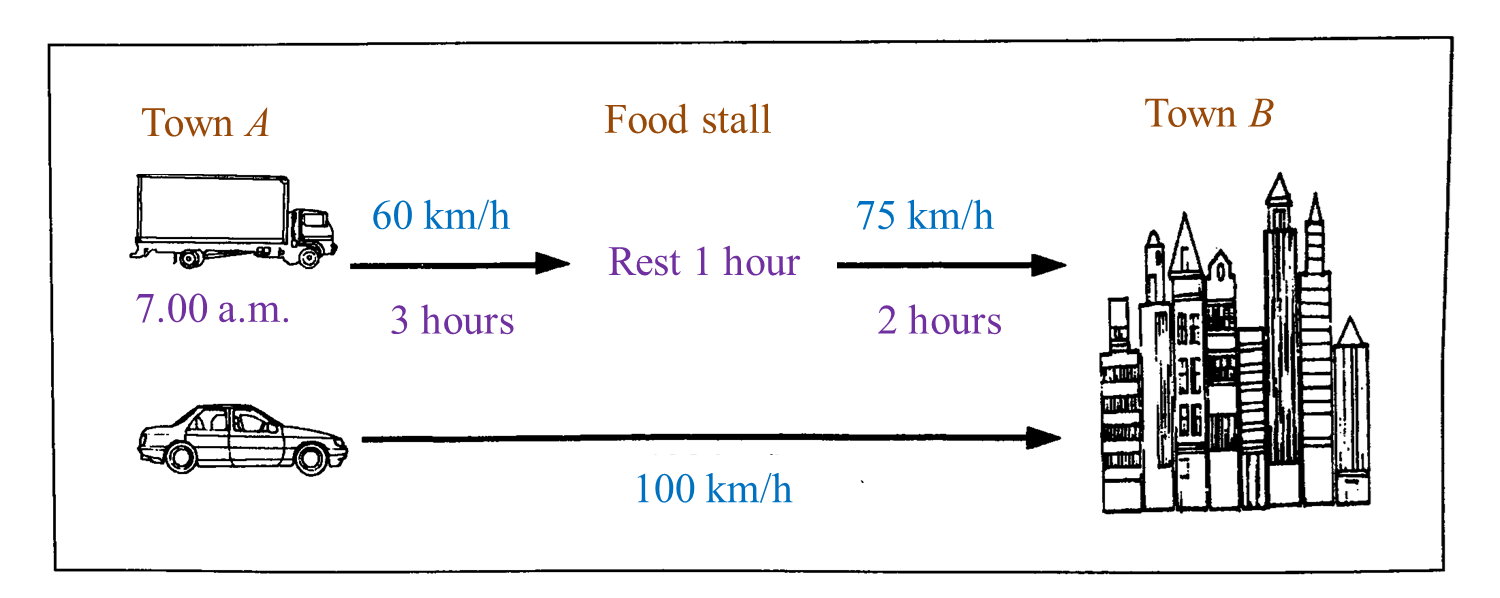(a) Jason started his journey from Town A at 7.00 a.m.
State the time, Jason reached at Town B.
(b) If both of them reached Town B at the same time, state the time Mary started her journey from Town A.

Solution:
(a)
Total time taken from Town A to Town B
= 3 hours + 1 hour + 2 hours
= 6 hours

Time Jason reached Town B
= 0700 + 0600
= 1300 hours → 1.00 p.m.

(b)# 14.2.2 Ratio, Rates and Proportions II, PT3 Focus Practice

Question 6:
Mei Ling drives her car from Kuantan to Kuala Terengganu for a distance of 170 km for 2 hours. She then continues her journey to Kota Bahru and increases her speed to 100 kmh-1 for 45 minutes.
Calculate the acceleration, in kmh-2, of the car.

Solution:

Question 7:
Diagram shows the distance between K and L.A car moves from K to L with an average speed of 80 kmh-1. After rest for 1 hour 30 minutes, the car then returns to K. The average speed of the car from L to K increases 20%. If the car reaches K at 5 p.m., calculate the time the car starts its journey from K.

SolutionThe car starts its journey from K at 11:50 a.m.

Question 8:
Mr Wong is going to watch a movie at 2.30 p.m at a cinema that is 60 km away from his house. He leaves at 1.25 p.m and drives at an average speed of 70 km/h for half an hour. If he drives at an average speed of 75 km/h for the remaining journey, will he arrive before the movie starts?